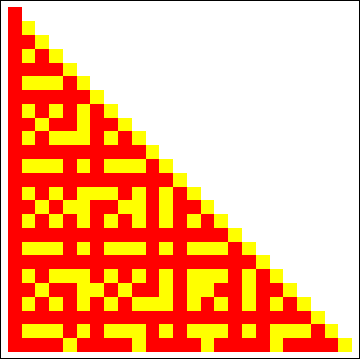## Sunday, 11 July 2010

### TOTIENT CARNIVALEuler's totient function: $latex \phi(n)$ is defined as the number of positive integers less than or equal to $latex n$, that are coprime to $latex n$, and using Iverson bracket, $latex \phi$ can be written as: (See reference )

$latex \phi(n) =\sum_{i=1}^{n}{[gcd(i,n)=1]}$

MULTIPLICATIVE BUT NOT COMPLETELY MULTIPLICATIVE FUNCTIONS:

The above summatory can be expressed with the aid of non completely multiplicative arithmetical funcions, using the fact that, some functions hold for inequalities similar to this one:

$latex f(n \cdot m) < f(n) \cdot f(m);$ if $latex gcd(n,m)\neq 1$ And that also hold for the properties that any multiplicative function has: $latex f(n)>0$

$latex f(n \cdot m) = f(n) \cdot f(m);$ if $latex gcd(n,m)= 1$

Then:

$latex \phi(n)=\sum_{i=1}^{n}{\bigg\lfloor \frac{f(i \cdot n)}{f(i)\cdot \f(n)}\bigg\rfloor}$

And applying this idea to arithmetical functions of common use, we can give, for the divisor sigma functions, the Piltz divisor functions and the squarefree kernel , respectively:

$latex \phi(n)=\sum_{i=1}^{n}{\bigg\lfloor \frac{\sigma_{k}(i \cdot n)}{\sigma_{k}(i)\cdot \sigma_{k}(n)}\bigg\rfloor}$

$latex \phi(n)=\sum_{i=1}^{n}{\bigg\lfloor \frac{\tau_{k}(i \cdot n)}{\tau_{k}(i)\cdot \tau_{k}(n)}\bigg\rfloor}$

$latex \phi(n)=\sum_{i=1}^{n}{\bigg\lfloor \frac{rad(i \cdot n)}{rad(i)\cdot rad(n)}\bigg\rfloor}$

Or we can construct an implicit formula for the Totient function:

$latex \phi(n)=\sum_{i=1}^{n}{\bigg\lfloor \frac {\phi(i)\cdot \phi(n)}{\phi(i \cdot n)}\bigg\rfloor}$

But we must take care that now:

$latex f(n \cdot m) > f(n) \cdot f(m);$ if $latex gcd(n,m)\neq 1$

so we must invert the fraction inside the floor function.

ADDITIVE BUT NOT COMPLETELY ADDITIVE FUNCTIONS:

The same thing can be done with additive but not completely additive functions:

$latex \phi(n)=\sum_{i=1}^{n}{\bigg\lfloor \frac{\omega(i \cdot n)}{\omega(i) + \omega(n)}\bigg\rfloor} \; (n>1)$

OTHER FORMULAS:

This way of constructing new formulas, seems to be trivial and useless, but this is only an excuse to show how works the characteristic or indicator functions, that are underneath the behaviour of the arithmetical functions, and thus the basic Set Theory inside Number Theory.

And of course we can extend this collection of formulas to other functions distinct than $latex \phi$, like for example $latex \pi$ the Prime Counting Function:

$latex \pi(n)=\sum_{i=2}^{n}{\bigg\lfloor \frac{i+1}{\sigma_{1}(i)}\bigg\rfloor} \; (n>1)$

$latex \pi(n)=\sum_{i=2}^{n}{\bigg\lfloor \frac{\phi(i)}{i-1}\bigg\rfloor} \; (n>1)$

$latex \pi(n)=\sum_{i=2}^{n}{\bigg\lfloor \frac{2}{\tau(i)}\bigg\rfloor}=\sum_{i=2}^{n}{\bigg\lfloor \frac{k}{\tau_{k}(i)}\bigg\rfloor} \; (n>1)$

Archives:

References:

-Peter Luschny - OEIS-Wiki: Sequences related to Euler's totient function.
- N. J. A. Sloane, The On-Line Encyclopedia of Integer Sequences.
A000720: pi(n), the number of primes <= n. Sometimes called PrimePi(n) to distinguish it from the number 3.14159... - N. J. A. Sloane, The On-Line Encyclopedia of Integer Sequences.
A000010: Euler totient function phi(n): count numbers <= n and prime to n. - R. Muller, The On-Line Encyclopedia of Integer Sequences.
A007947: Largest squarefree number dividing n (the squarefree kernel of n)